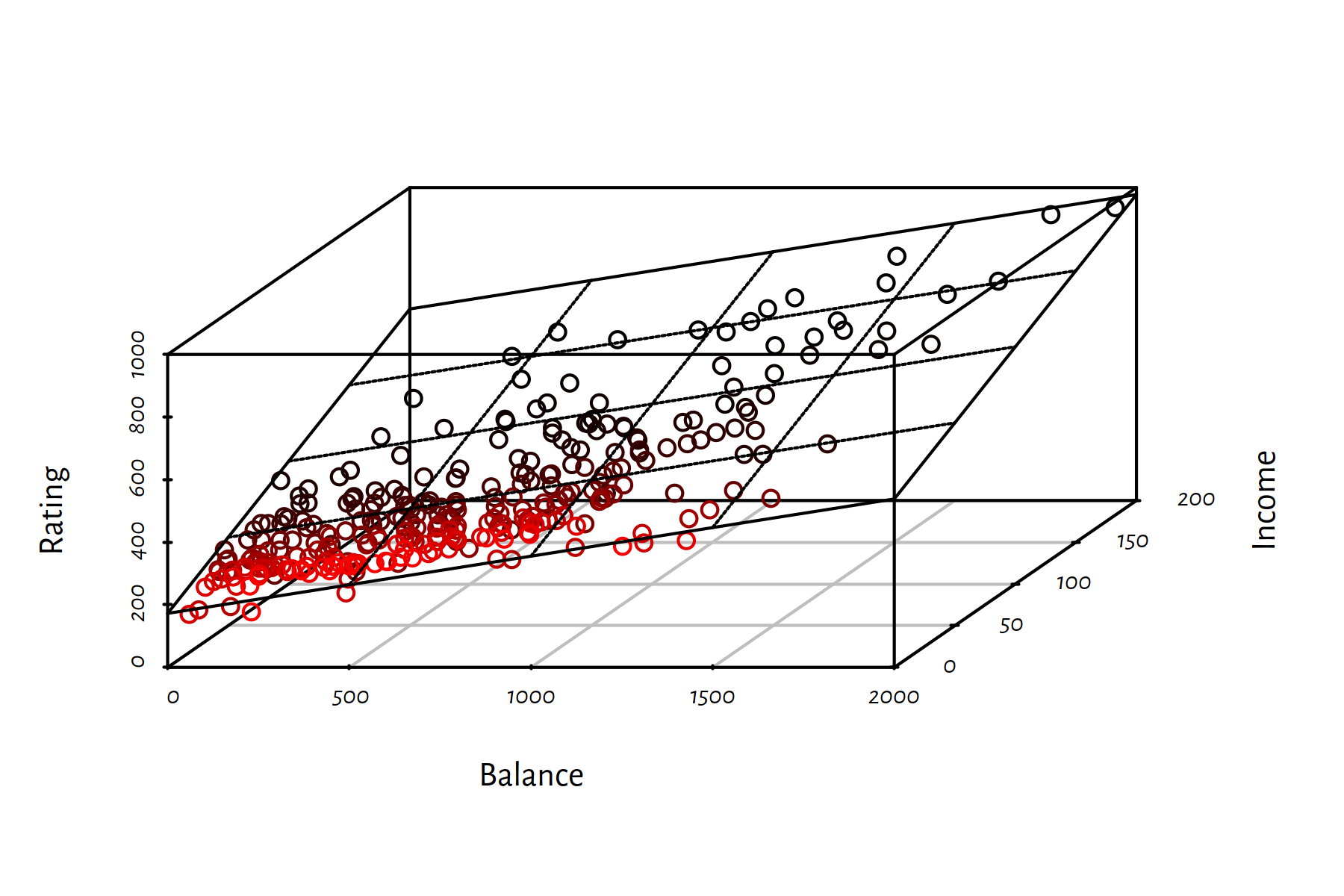## 2.2 Multiple Linear Regression

### 2.2.1 Problem Formulation

Let’s now generalise the above to the case of many variables $$X_1, \dots, X_p$$.

We wish to model the dependent variable as a function of $$p$$ independent variables. $Y = f(X_1,\dots,X_p) \qquad (+\varepsilon)$

Restricting ourselves to the class of linear models, we have $Y = \beta_0 + \beta_1 X_1 + \beta_2 X_2 + \dots + \beta_p X_p.$

Above we studied the case where $$p=1$$ with $$\beta_1=a$$ and $$\beta_0=b$$.

The above equation defines:

• $$p=1$$ — a line
• $$p=2$$ — a plane
• $$p\ge 3$$ — a hyperplane

Most people find it difficult to imagine objects in high dimensions, but we are lucky to have this thing called maths.### 2.2.2 Fitting a Linear Model in R

lm() accepts a formula of the form Y~X1+X2+...+Xp.

It finds the least squares fit, i.e., solves $\min_{\beta_0, \beta_1,\dots, \beta_p\in\mathbb{R}} \sum_{i=1}^n \left( \beta_0 + \beta_1 x_{i,1}+\dots+\beta_p x_{i,p} - y_i \right) ^2$

X1 <- as.numeric(Credit$Balance[Credit$Balance>0])
X2 <- as.numeric(Credit$Income[Credit$Balance>0])
Y  <- as.numeric(Credit$Rating[Credit$Balance>0])
f <- lm(Y~X1+X2)
f$coefficients # ß0, ß1, ß2 ## (Intercept) X1 X2 ## 172.5586670 0.1828011 2.1976461 By the way, the above 3D scatter plot was generated by calling: par(mar=c(4, 4, 0.5, 0.5)) library("scatterplot3d") s3d <- scatterplot3d(X1, X2, Y, angle=60, # change angle to reveal more highlight.3d=TRUE, xlab="Balance", ylab="Income", zlab="Credit Rating", las=1) s3d$plane3d(f, lty.box="solid")

(s3d is an R list, one of its elements named plane3d is a function object – this is legal)